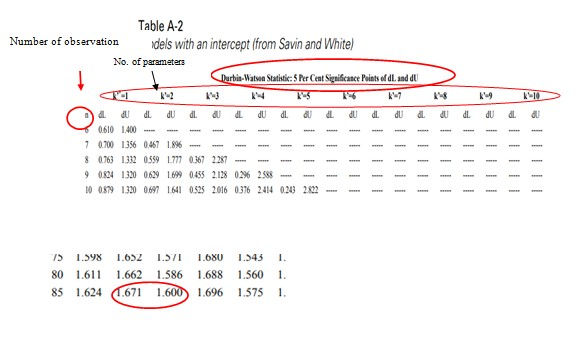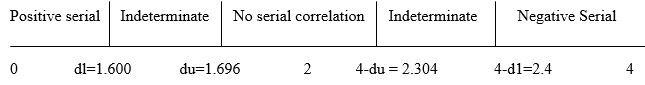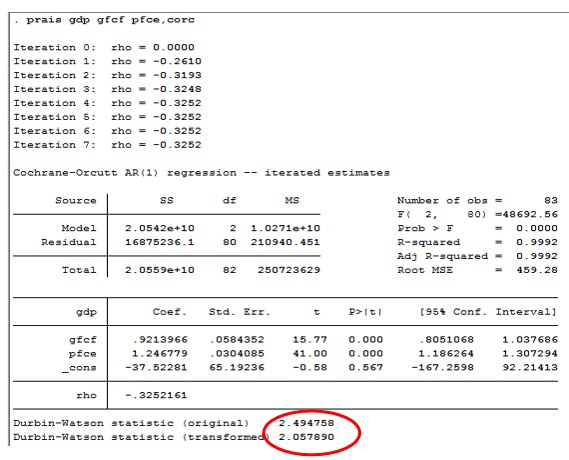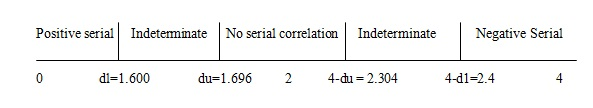# How to test time series autocorrelation in STATA?

The previous article showed how to perform heteroscedasticity tests of time series data in STATA. It also showed how to apply a correction for heteroscedasticity so as not to violate Ordinary Least Squares (OLS) assumption of constant variance of errors.  This article shows a testing serial correlation of errors or time series autocorrelation in STATA. Autocorrelation problem arises when error terms in a regression model correlate over time or are dependent on each other.

## Why test for autocorrelation?

It is one of the main assumptions of OLS estimator according to the Gauss-Markov theorem that in a regression model:

```Cov(ϵ_(i,) ϵ_j )=0 ∀i,j,i≠j,
where Cov is the covariance and ϵ is the residual.```

Presence of autocorrelation in the data causes and to correlate with each other and violate the assumption, showing bias in OLS estimator. It is therefore important to test for autocorrelation and apply corrective measures if it is present. This article focuses on two common tests for autocorrelation; Durbin Watson D test and Breusch Godfrey LM test. Like the previous article (Heteroscedasticity test in STATA for time series data), first run the regression with the same three variables Gross Domestic Product (GDP), Private Final Consumption (PFC) and Gross Fixed Capital Formation (GFC) for the time period 1997 to 2018.

## Durbin Watson test for autocorrelation

Durbin Watson test depends upon 2 quantities; the number of observations and number of parameters to test. In the dataset, the number of observations is 84 and the number of parameters is 2 (GFC and PFC). In the Durbin Watson table two numbers are present– dl and du. These are the “critical values” (figure below).Figure 1: Critical values of Durbin Watson test for testing autocorrelation in STATA

Durbin Watson statistic ranges from 0 to 4. As the above scale shows, statistics value between 0 to dl represents positive serial autocorrelation. Values between dl and du; 4-du and 4-dl indicate serial correlation cannot be determined. The value between du and 4-du represents no autocorrelation. Finally, the value between 4-dl and 4 indicates negative serial correlation at 95% confidence interval.

Command for Durbin Watson test is as follows:

`dwstat`

However, STATA does not provide the corresponding p-value. To obtain the Durbin Watson test statistics from the table conclude whether the serial correlation exists or not. Download the Durbin Watson D table here.Figure 2: Durbin Watson test statistics table for testing autocorrelation in STATA

In the above figure, the rows show the number of observations and the columns represents “k” number of parameters. Here the number of parameters is 2 and the number of observations is 84. Consequently:

Durbin Watson lower limit from the table (dl) = 1.600

Durbin Watson upper limit from the table (du) = 1.696

Therefore, when du and dl are plotted on the scale, results are as follows (figure below).Figure 3: Results of Durbin Watson test

Durbin Watson d statistics from the STATA command is 2.494, which lies between 4-dl and 4, implying there is a negative serial correlation between the residuals in the model.

## Breusch-Godfrey LM test for autocorrelation

Breusch-Godfrey LM test has an advantage over classical Durbin Watson D test. The Durbin Watson test relies upon the assumption that the distribution of residuals are normal whereas Breusch-Godfrey LM test is less sensitive to this assumption. Another advantage of this test is that it allows researchers to test for serial correlation through a number of lags besides one lag that is a correlation between the residuals between time t and t-k (where k is the number of lags). This is unlike the Durbin Watson test which allows testing for only correlation between t and t-1. Therefore if k is 1, then the results of Breusch-Godfrey test and Durbin Watson test will be the same.

Follow the below command for Breusch Godfrey LM test in STATA.

`estat bgodfrey`

The following results will appear as shown below.Figure 4: Results of Breusch-Godfrey LM test for autocorrelation in STATA

The hypothesis in this case is:

• Null hypothesis: There is no serial correlation.
• Alternative Hypothesis: There is a serial correlation.

Since from the above table, chi2 is less than 0.05 or 5%, the null hypothesis can be rejected. In other words, there is a serial correlation between the residuals in the model. Therefore correct for the violation of the assumption of no serial correlation.

## Correction for autocorrelation

To correct the autocorrelation problem, use the ‘prais’ command instead of regression (same as when running regression), and the ‘corc’ command at last after the names of the variables.

Below is the command for correcting autocorrelation.

`prais gdp gfcf pfce, corc`

The below results will appear .Figure 3: Regression results with correction of autocorrelation in STATA

At the end of the results, finally, calculate original and new Durbin Watson statistics as follows.Figure 4: Calculation of original and new Durbin Watson statistics for autocorrelation in STATA

New D-W statistic value is 2.0578 which lies between du and 4-du, implying that there is no autocorrelation now. Thus it has been corrected.

Furthermore, the next article discusses the issue of multicollinearity. Multicollinearity arises when two or more than two explanatory variables in the regression model highly correlate with each other.

### Rashmi Sajwan

Research Analyst at Project Guru
Rashmi has completed her bachelors in Economic (hons.) from Delhi University and Masters in economics from Guru Gobind Singh Indrapastha University. She has good understanding of statistical softwares like STATA, SPSS and E-views. She worked as a Research Intern at CIMMYT international maize and wheat improvement centre. She has an analytical mind and can spend her whole day on data analysis. Being a poetry lover, she likes to write and read poems. In her spare time, she loves to do Dance.

#### Latest posts by Rashmi Sajwan (see all)

Discussions

1.Khainga